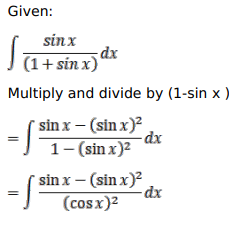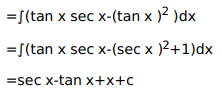# Mark against the correct answer in each of the following:

Question:

Mark $(\sqrt{)}$ against the correct answer in each of the following:

$\int \frac{\sin x}{(1+\sin x)} d x=?$

A. $\sec x+\tan x+x+C$

B. $\sec x-\tan x+x+C$

C. $-\sec x+\tan x+x+C$

D. None of these

Solution: